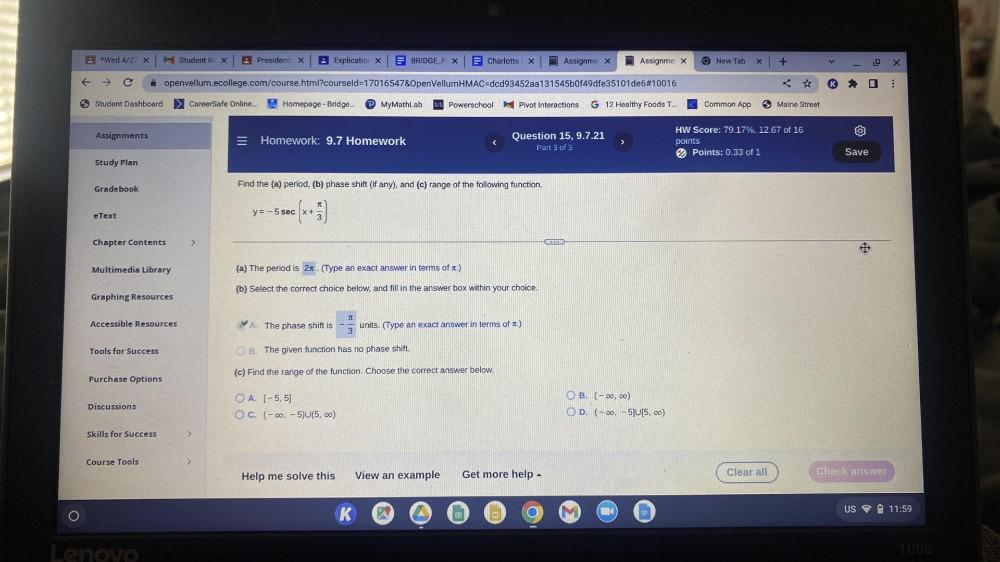Question:

# Find the (a) period, (b) phase shift (if any), and (c) range of the following function. y = -5 sec[x + π/3 ] (a) The period is 2π. (Type an exact answer in terms of π.) (b) Select the correct choiceFind the (a) period, (b) phase shift (if any), and (c) range of the following function. y = -5 sec[x + π/3 ] (a) The period is 2π. (Type an exact answer in terms of π.) (b) Select the correct choice below, and fill in the answer box within your choice. (A) The phase shift is -π/3 units. (Type an exact answer in terms of π.) (B) The given function has no phase shift. (c) Find the range of the function. Choose the correct answer below. (A) [-5,5] (B) (-∞, ∞) (C) (-∞, -5)U(5, ∞) (D) (-∞, -5]U[5, ∞)